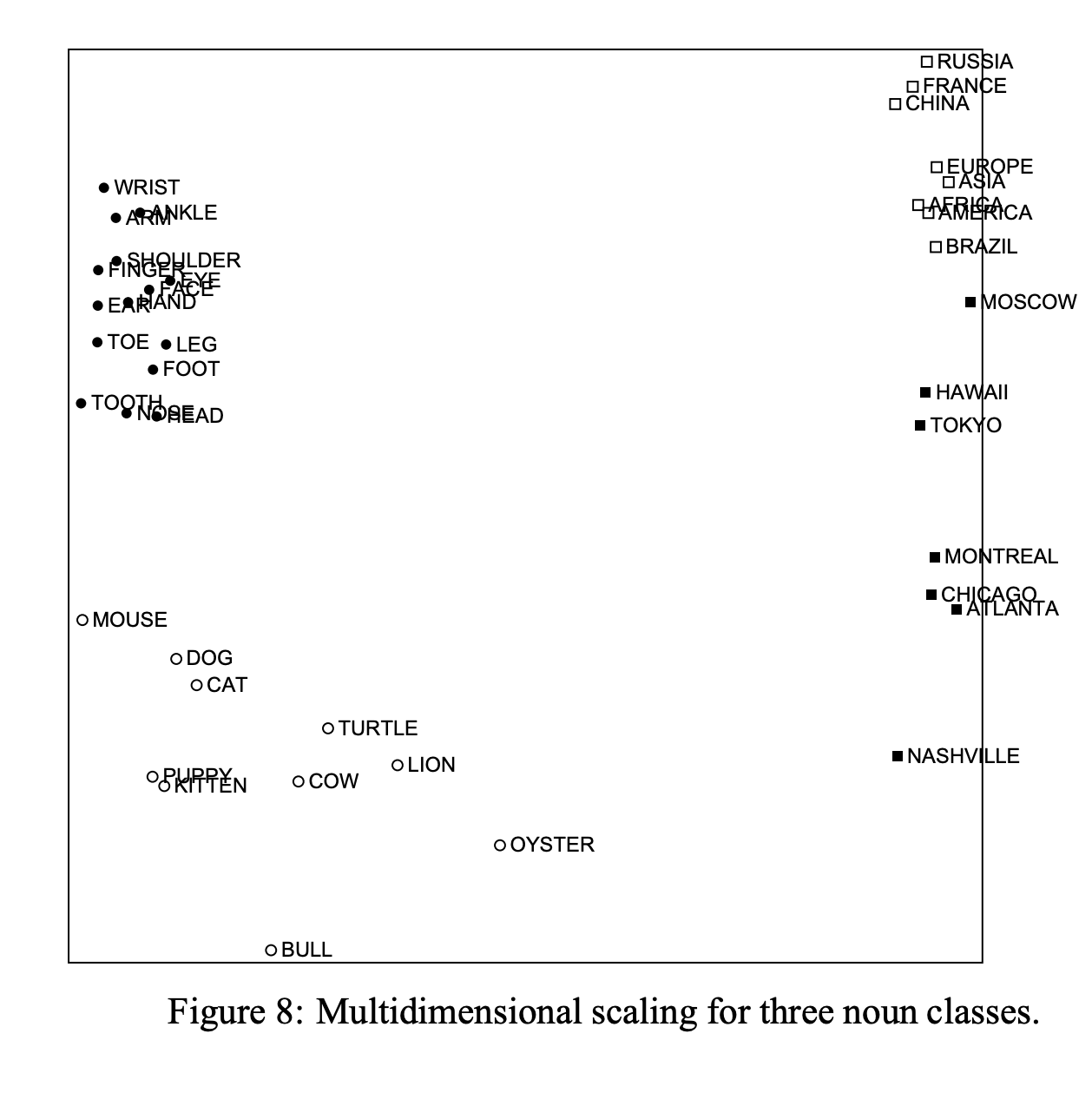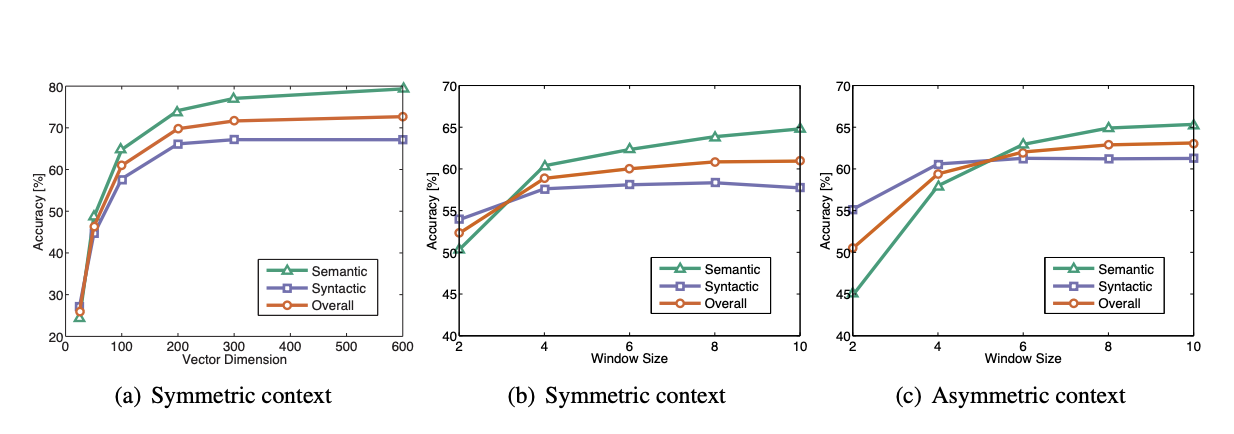# CS224N: NLP with Deep Learning | Winter2019 | Lecture2

Jun 05, 2020 · 5 mins readShare this

Images: Vector directions related to word classes (Rohde et al. 2005)

# Word Vectors and Word Senses

• Finish last time’s lecture

(showed more examples)

and we can see the problem it can’t represent the polysemy

drawed PCA: remember this way we lose lots of information because we just chose first two principle components

purpose of this class is just end of the course you can read paper(from classical to contemporary), and understand them

• what is parameter of word2vec

Only two, input vector and output vector

each vector is represented as ROW (at almost all of modern ML library)

We are going with just one probability -> same prediction at each point.

quite interesting cz model is so simple and works well

• Tip: Function word has fix high probability, (they are closed) so removing it results in better Word Vectors 1

• Brief explain of optimization

actual loss function would be more complex, bumpy, not convex

• Problem of this model and Stochastic Gradient Descent
1. our objective function has to deal with every each element of corpora.
2. Nobody uses this since cost efficiency is very horrible
3. So we use SGD (not just one data but with group, which is called batch)
• Shortage of SGD

Tends to end up sparse distribution, so that usually use (probability) smoothing.

• Details of Word2vec

Why do we learn two vectors ? M: effective, easy to partial derivative. if we use just one parameter, math becomes more difficult, but practically you average it.

• skip-grams/CBOWs
• Naive softmax is slow because it use all the vocabulary.
Idea: Train Binary Logistic Regression
1. numerator : actually observed, give high prob
2. denominator : noise, which was randomly selected.

HW2: The skip-gram model with negative sampling
k would be anything the size of sample you want to choose
$$P(w) = \frac{U(w)^{\frac{3}{4}}}{Z}$$

• It comes out that this experiment is not that replicable(which needs lots of hyper-parameter, tricks)

• randomly select the batches in corpus, for each iteration, not ordering and sequencing, and this also saves some memory.

• why not use n-gram, window-based co-occurrence matrix, and this became sparse matrix -> occupy so much bigger space, not that robust. -> then what about other dimension reduction methods?

(HW1) SVD, factorize the matrix</br>results: least square error in estimation
this way we can also make word-vectors

### Can’t we approach to build model using frequency? - Glove

1. Remove too much frequent words which is function words
2. weigh more where it is closer
3. Use Pearson correlation instead of counts, then set negative values to 0

( And this techs were used in word2vec)

Sort of direction in vector spaces matches the word’s feature, and below pic shows it matches with POS, in this case verb and noun

And this is meaningful because this proved constructed VS does well in analogy.

Conventional methods also can give you good vectors.

And this could be the origin of Glove

• Count based vs direct based.
Direct based model goes sample by sample so that can’t use that well the statistics
On the other hand, Count based model(usually classical model) can use stats more efficiently and also the memory.

• Encoding meaning in vector differences (= fraction of log) Using co-occurence
Insight: Ratio of co-occurrence probabilities can encode meaning components. (not enough just co-occurrence!)

Q. How can we capture ratios of co-occurrence probabilities as linear meaning components in a word vector space?

Dot product should become similar as much as possible with log of co-occurrence(#TODO1: check again, can’t understand)

### How to evaluate word vectors?

• intrinsic vs extrinsic(use in real system(=real application) e.x. QA, web search…)

intrinsic ex: calculate cosine, and see if it matches with language intuition

(Tot. means analogy)

• Comparing using hyper parameter (i.e., Vector dimensions, Window sizes)if you only use context which is at one side, that is not as good as using both sided matrix (#TODO2: check the codes)

• On the Dimensionality of Word Embedding

mathy ideas using matrix perturbation idea.
=> if you increase dimensions, the performance gets flatten and they proved using perturbation theory (??)

• much time helps, and wikipedia is better than news data (1.6b wiki data is better than 4.3b of news data in web) when making word vectors

• WordSim353: Human judgement, which was from psycology

### More problem regarding word senses (Ambiguity, Polysemy)

• Most words have lots of meaning - ex. pike
• common words
• existed for a long time

Actually, this argument was existed when word2vec came out, with labelling polysemy(multiple senses) and embeds it. (Huang et al. 2012), and cluster the departed words
=> but with this method, the senses are not that clear.

• Linear Algebraic Structure of Word Senses, with Application to Polysemy (Arora, Ma, TACL2018)

• WHY nlp people are mad at Word2Vec idea?

• It came out that good word2vec directly related with subtask(extrinsic tasks) enhancement (like, name entity)
1. see the paper of Sanjeev Arora’s group / ???